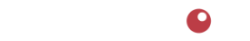# Cost-Price-Margin Calculator

This calculator determines the profit margin % of a product when both cost and price are known.

Calculating profit margin % is a useful tool in making business decisions and is common in the food industry.

Profit margin % is defined as gross profit expressed as a percentage of selling price. It is calculated by dividing gross profit (price - cost) by price.

Profit margin % calculations are useful when analyzing profitablity of two or more products when their costs and/or prices differ. That's because the results are reported as a percentage rather than in raw numbers.

To use this calculator, enter the cost and price of the food product. Then press the [Calculate] button.
The calculated profit margin % will appear in the space labeled "Margin %".

Be sure to check out all our calculators located here.

### Margin % Calculator

Explanation:

Be sure to check out all our calculators located here.4110 SE Hawthorne Blvd. #739

Portland OR 97214

503.234.7199

Notice | Privacy